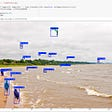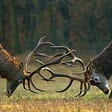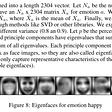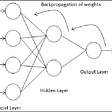# Univariate Linear Regression

## An introduction to machine learning.

In machine learning, there are two main algorithms to get your computer to act smart: supervised and unsupervised learning.

In supervised learning, the computer is given data about the subject usually being inputs and outputs. The most common use of supervised learning is to classify objects. For example, I might give my computer a set of pictures of dogs and I want it to output 0 for Pekingese, 1 for Golden Retriever, and 2 for German Shepherd. To train this computer to recognize the dogs, I would give it millions of dog pictures with their respective numbers attached to each image. The computer would then learn, by the use of some ML algorithm, to associate pixel patterns with a certain breed of dog.

In unsupervised learning, the computer is given data that has no labels. An unsupervised learning algorithm has to find structure in structure-less data. The most common tasks within unsupervised learning are clustering, representation learning, and density estimation. The following picture depicts the idea of clustering in which the computer takes images of cartoons and clusters them into their respective cartoon styles.

Just an FYI, linear regression is a supervised learning algorithm. Because of this, I will give some information about how some of the features of this algorithm will be represented mathematically.

In supervised learning and linear regression, you are always given a “training set” to teach the ML algorithm how to classify new data.

Here are some variables and what they mean in context of training samples:

m = number of training examples
x = “input” variable/feature
y = “output” variable/target
(x,y) = one training sample (input, output)
(xⁱ,yⁱ) = iᵀᴴ training sample

DON’T WORRY (if you are worrying)! This will all make tons more sense in a bit.

Here is an example of how these variables can be used.

Let’s say that you want to teach the computer to guess a house’s price based on its size. Well, you would first have to give the algorithm a set of training samples that contain many different house sizes and prices. Because we want to give the computer a size and have it guess the price, the size is the input and the price is the output. Or, in math words, the size is x (input) and the price is y (output). Here is an example of what the training data would look like (please note that in reality, there would have to be many many more training samples to get accurate results):

Now let’s match some variables to this data set.

m = 5 because there are 5 training samples (denoted by i)
x=1130 because it is the 3rd-row size
(x, y)=(860,50000) because it is the fourth-row x and y values

Hopefully, that clears up some complexities with the math representations.

# Linear Regression

In linear regression, we use a training set to come up with an algorithm that creates a function “h” that maps x to y. In the housing prices example, we need to use that data to come up with a function that maps the size of houses to their price (x to y, inputs to outputs).

The function that we are trying to develop looks like this:

This should look fairly familiar, right? Doesn’t it resemble a line in slope-intercept form?

That is because linear regression is essentially the algorithm for finding the line of best fit for a set of data. Using the house data above, the graph below depicts the plotted training samples and the function (dotted line) that linear regression would produce for the data.

Let’s take a look at that representation of the function:

The algorithm finds the values for 𝜃₀ and 𝜃₁ that best fit the inputs and outputs given to the algorithm. This is called univariate linear regression because the 𝜃 parameters only go up to 1. The univariate linear regression algorithm is much simpler than the one for multivariate. The function that multivariate linear regression produces looks like this:

I plan to write an article on multivariate linear regression soon-ish.

This next piece of math I will show you is a way to find the average of the difference between the predicted values/outputs and the actual values/outputs. Given a number for 𝜃₀ and 𝜃₁ each, this function will essentially show us, how “wrong” the function h(x) is. Another way to say this is how bad values were chosen for 𝜃₀ and 𝜃₁. Here is the expression:

Let’s break this down.

m = # of training examples
hᶿ(xⁱ) = the predicted value. Remember, h(x) is the function the algorithm is developing (the line of best fit), so the return value from that function is a “prediction”.
yⁱ = actual output from training samples.

Here is the goal for this expression: Find the numbers 𝜃₀ and 𝜃₁ such that the average of the sum of the predicted value, h(x), minus the actual value is minimized (as small as possible).

This expression is called the “cost function”. It defines how much error there is in the parameter values we chose. The one defined above is known as the “squared error function”. It is the most commonly used cost function for linear regression problems. Cost functions are typically denoted using J as the name of the function.

# Univariate Linear Regression Review

Hypothesis:

Parameters:

Cost Function:

Goal:

Minimize J(𝜃₀,𝜃₁) by choosing the optimal values for 𝜃₀ and 𝜃₁. This way, when a new x value is inputted into the function h(x), the returned value y will hopefully be an accurate pair to the inputted variable.

Please note that the cost function J(𝜃₀,𝜃₁) for two variables can be visualized in a 3d plane looking something like this:

It looks like a mountain range! The hight of the mountain from 0 at any given point represents the squared error. The areas in the depiction that are high up represent parameter values that cause the squared error to be high. Likewise, valleys represent optimal parameter values because they make the squared error small in value.

Now that you know the function and parameters we are trying to optimize (h(x)) and the cost function that tells us how “wrong” we are (how much error there is), it is time to learn exactly HOW to optimize these parameters 𝜃₀ and 𝜃₁ such that the cost function is minimal (as close as possible to 0). The main algorithm to optimize these parameters and minimize the cost function result is called Gradient Descent. If you look once more at the image above, the valleys are the parameter values that gradient descent finds. It can be compared to rolling a ball down a hill and the place where it comes to rest holds the optimal parameter values. In laymen terms, that is how Gradient Descent finds the optimal values — “rolling the ball down the hill”.

--

--

--

## More from The Startup

Get smarter at building your thing. Follow to join The Startup’s +8 million monthly readers & +756K followers.

## How to Solve Sudoku with Convolutional Neural Networks (CNN).## I am the first Machine Learning Engineer, now what?## How to Train Yolo v3 to Detect Custom Objects with Free GPU## Loan Data Machine Learning Model## Difficult stuff in simple words: Data Science vs Machine Learning vs Artificial intelligence## Reconstruct competitor pricing using Machine Learning (GBM) in R## Emotion Recognition using Eigenface/PCA Reconstruction## Let’s Learn: Neural Nets #4 — Weights and Biases## BackPropagation Neural Networks- Classification and Regression from scratch with python## Linear Classification in Machine Learning## Machine Learning